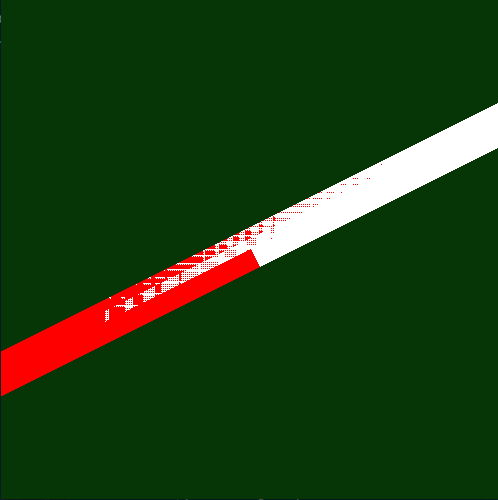# Geometry Shader - Texture Coordinates Interpolation issue

Hello Everyone,
For Last few days i am working on Geometry Shaders to render Thicklines
My Question is about the “Texture Coordinate Interpolation” when used with Geometry Shader.

Just See This Image (You will understand what i am trying to do…)

–> I used VBOs/ VAOs
–> used GL_LINE
–> I used simple vertex data(x,y,z) Points{A,B}
`float vertices[]={ -0.2f,-0.1f,0.0f, 0.2f,0.1f,0.0f}`

–> And This is my Shader File( Vertex/Geometry/Fragment)

`````` #shader vertex

#version 330 core
layout (location = 0) in vec3 pos;
uniform mat4 u_MVP;

void main()
{
gl_Position = u_MVP *vec4(pos, 1.0);
}

//############################################################

#version 330 core
layout (lines) in;
layout (triangle_strip, max_vertices = 4) out;

out vec2 TXC;

uniform float   u_W;                     //Width of Line(pixels)
uniform vec2    WIN_SCALE;    //window Height,Width

vec2 screen_space(vec4 vertex)
{
return vec2( vertex.xy /vertex.w ) * (WIN_SCALE/2.0f);
}

void main(void)
{
float THICKNESS=u_W/2.0f;

vec2 p1 = screen_space(gl_in.gl_Position );

vec2 p2 = screen_space(gl_in.gl_Position);

vec2 v1 = normalize(p2-p1);

vec2 n1 = vec2(-v1.y, v1.x);  //Normal

TXC=vec2( 0,1);
gl_Position = vec4( 2.0f*(p1 + THICKNESS*n1) / WIN_SCALE, 0.0, 1.0 );
EmitVertex();

TXC=vec2(0,0);
gl_Position = vec4( 2.0f*(p1 - THICKNESS*n1) / WIN_SCALE, 0.0, 1.0 );
EmitVertex();

TXC=vec2(1,1);
gl_Position = vec4( 2.0f*(p2 + THICKNESS*n1) / WIN_SCALE, 0.0, 1.0 );
EmitVertex();

TXC=vec2(1,0);
gl_Position = vec4( 2.0f*(p2 - THICKNESS*n1) / WIN_SCALE, 0.0, 1.0 );
EmitVertex();

EndPrimitive();
}
//############################################################

#version 330 core

in vec2 TXC;

void main()
{
float u=TXC.x;              // u or x coordinate of current fragment

if(u<0.5)
gl_FragColor = vec4(1,0,0,1);   //RED

else
gl_FragColor = vec4(1,1,1,1);   //WHITE

}
``````

Now The RESULTS–
–> Initially i got this… Good. I am OK with this

–> NOW THE ISSUE
When I Zoom It Very very much , I saw Breakings in the rendering…
Like this…The interpolated value of tex.u in fragment shader doest seems accurate…

1. Am I doing Something Wrong Here???
2. So Is there any solution for this???

One thing more is that…This thing happens much on AMD platform , On NVIDIA it looks fine(atleast where i tested)

And this is segment of detail problem( i want to keep it short)
Please Help…Note: I am not using any Texture here, i am just using tex coordinates to use it for creating colors/gaps in rendering